# Gradient backward, Chain Rule, Refactoring

Mar 02, 2020 · 14 mins read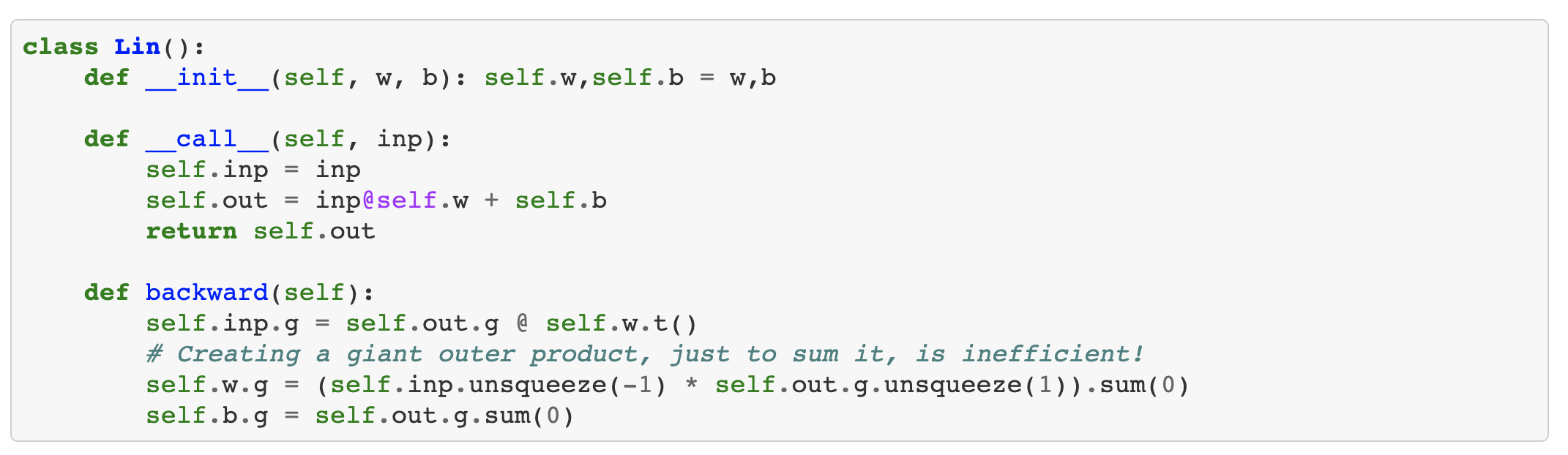Share this
• This note is divided into 4 section.

” Lecture 08 - Deep Learning From Foundations-part2 “

### CONTENTS

• Forward process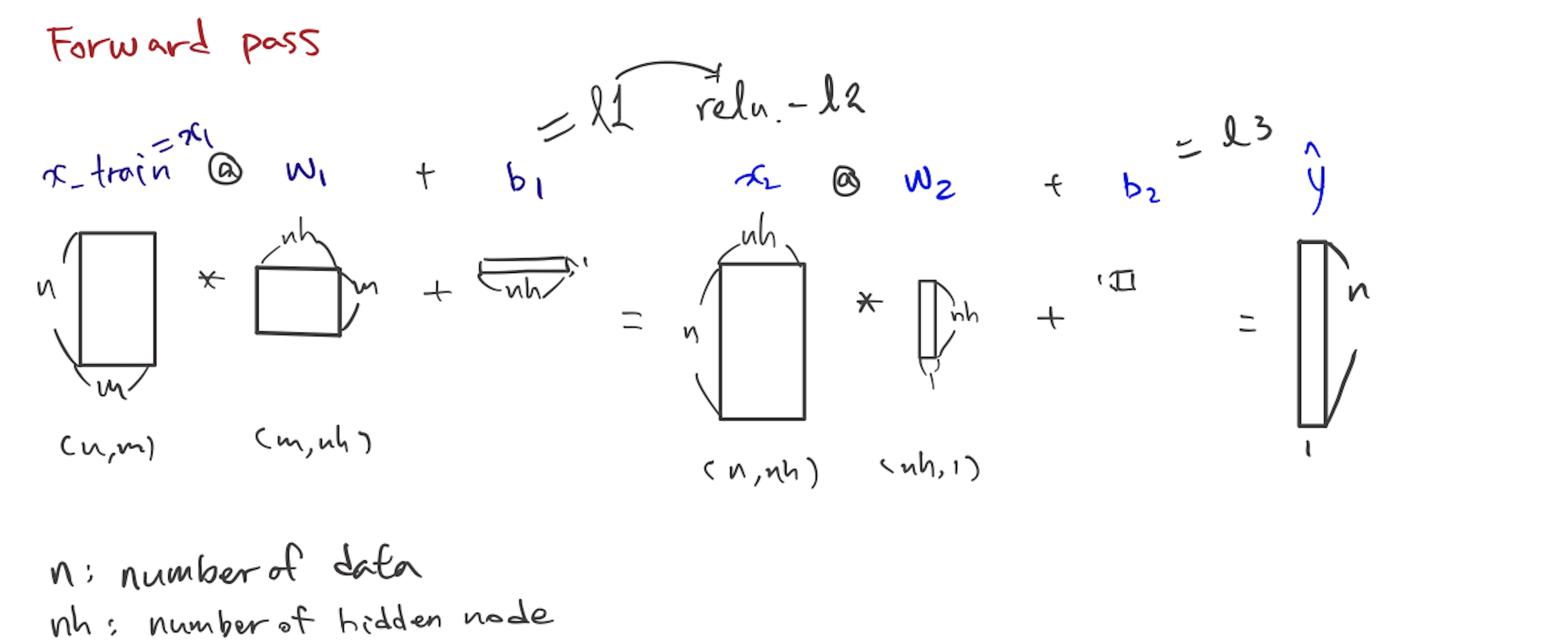### Foundation version

#### Gradients backward pass

• Gradients is output with respect to parameter
• we’ve done this work in this path(below)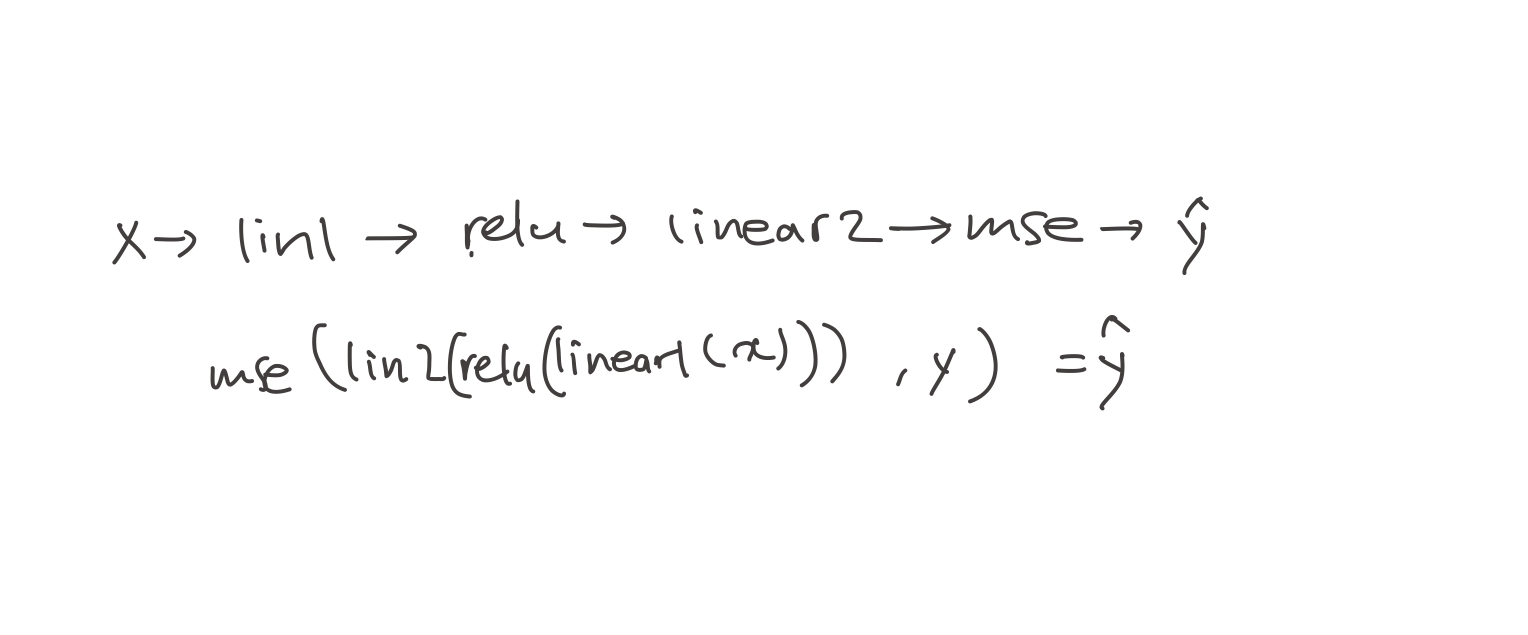• to simplify this calculus, we can just change it into

$$y=f(u)$$, $$u=g(x)$$

• So, you should know of the derivative of each bit on its own, and then you multiply them all together. As a result, it would be $dy$ over $dx$ cross over the data.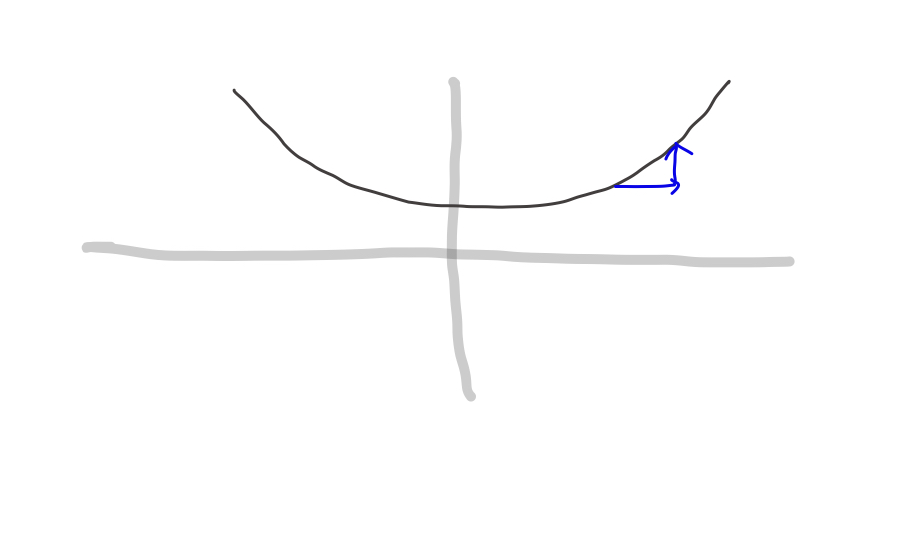• So you can get gradient, output with respect to parameter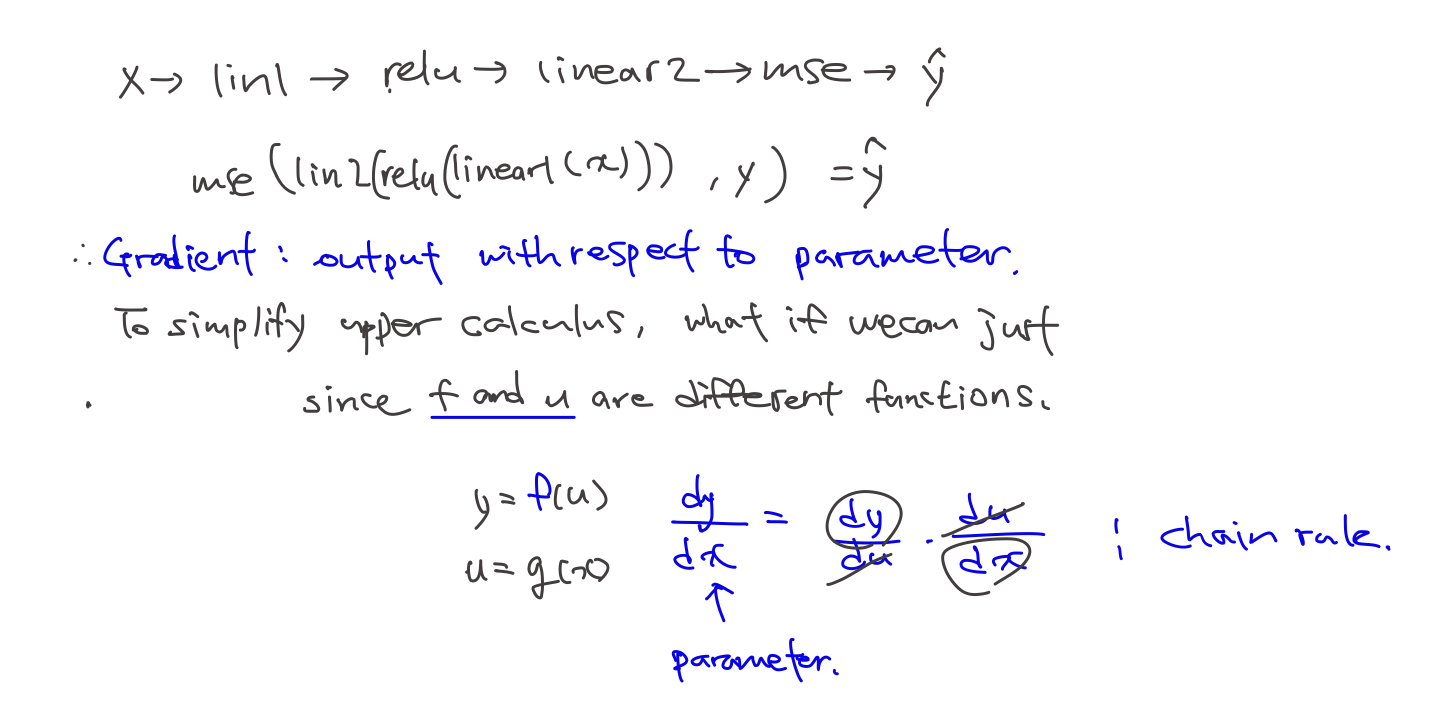• What order should we calculate?BTW, why Jeremy wrote $\hat y$, not Loss function?1

##### decompose function
• We want to get derivative of $w_1$ which forms $t=x_1 @ w_1 + b_1$
• But, we have a estimation of answer (we call it y hat) now
• So, I will decompose funciton to trace target variable.
$\overset{\rightharpoonup}{u}\ \ linear_1\ \ \overset{\rightharpoonup}{w}\ \ ReLU\ \ \overset{\rightharpoonup}{v}\ \ linear_2\ \ \overset{\rightharpoonup}{a}\ \ MSE = \hat y$
• Using the above forward pass, we can suppose some function from the end.
• start from $\hat y$, We know MSE funciton got two parameters, output, $u$ and target $y$.
• from MSE’s input we know $linear_2$ function’s output and supposing v is input of that function, $linear_2(v) = u$
• similarly, v became output of $ReLU, ReLU(t) = v$##### chain rule with code
• examplify backward process by random sampling

• To get a variable, I modified forward model a little

def model_ping(out = 'x_train'):
l1 = lin(x_train, w1, b1) # one linear layer
l2 = relu(l1) # one relu layer
l3 = lin(l2, w2, b2) # one more linear layer
return eval(out)

• Be careful we don’t use mse_loss in backward process

1) start with the very last function, which is loss funciton. MSE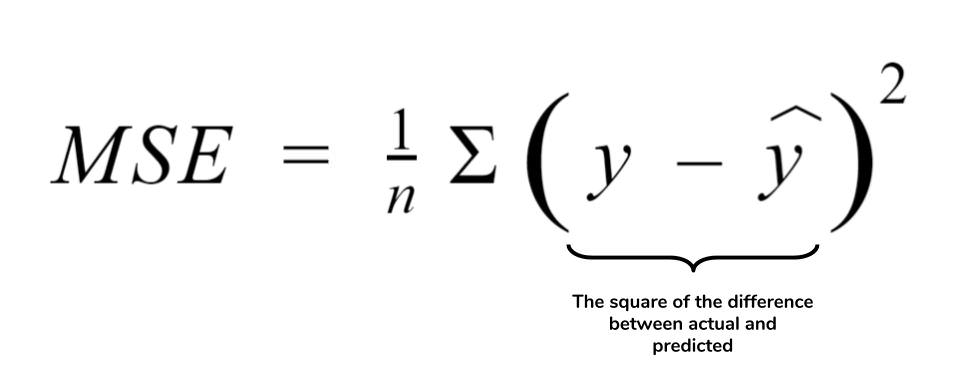$\color{red}{\frac{\partial}{\partial u}MSE(u,y)} \times\frac{\partial}{\partial v}l_2(v)\times\frac{\partial}{\partial t}ReLU(t)\times\frac{\partial}{\partial x}l_1(x)$
• If we codify this formula,
def mse_grad(inp, targ):  #mse_input(1000,1), mse_targ (1000,1)
# grad of loss with respect to output of previous layer
inp.g = 2. * (inp.squeeze() - targ).unsqueeze(-1) / inp.shape

• And, this can be examplified like below.
• Notice that input of gradient function is same with forward function
y_hat = model_ping('l3') #get value from forward model
y_hat.g = ((y_hat.squeeze(-1)-y_train).unsqueeze(-1))/y_hat.shape

y_hat.g.shape
>>> torch.Size([50000, 1])

• We can just calculate using broadcasting, not using squeeze. then why should do and unsqueeze again?
🎯 It’s related with random access memory(RAM).. If I don’t squeeze, (I’m using colab) it out of RAM.

2) Derivative of linear2 function

$\frac{\partial}{\partial u}MSE(u,y) \times\color{red}{\frac{\partial}{\partial v}l_2(v)} \times \frac {\partial}{\partial t}ReLU(t)\times\frac{\partial}{\partial x}l_1(x)$
• This process’s weight dimensions defined by axis=1, axis=2.
• axis=0 dimension means size of data. This will be summazed by .sum(0) method.
• unsqeeze(-1)&unsqeeze(1) seperates the dimension, and make a dot product, and vanish axis=0 dimension.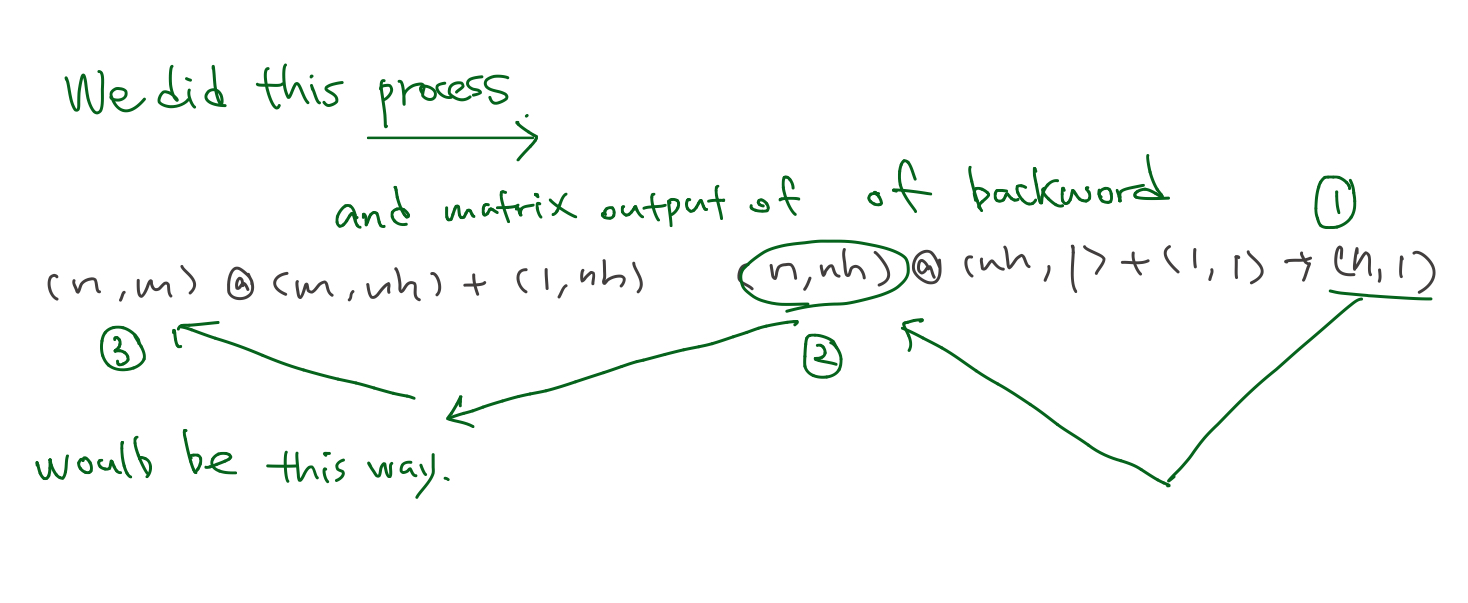def lin_grad(inp, out, w, b):
# grad of matmul with respect to input
inp.g = out.g @ w.t()
w.g = (inp.unsqueeze(-1) * out.g.unsqueeze(1)).sum(0)
b.g = out.g.sum(0)

• Examplified below
lin2 = model_ping('l2'); #get value from forward model
lin2.g = y_hat.g@w2.t();
w2.g = (lin2.unsqueeze(-1) * y_hat.g.unsqueeze(1)).sum(0);
b2.g = y_hat.g.sum(0);

lin2.g.shape, w2.g.shape, b2.g.shape
>>> torch.Size([50000, 50])torch.Size([50, 1])torch.Size()

• Notice going reverse order, we’re passing in gradient backward

3) derivative of ReLU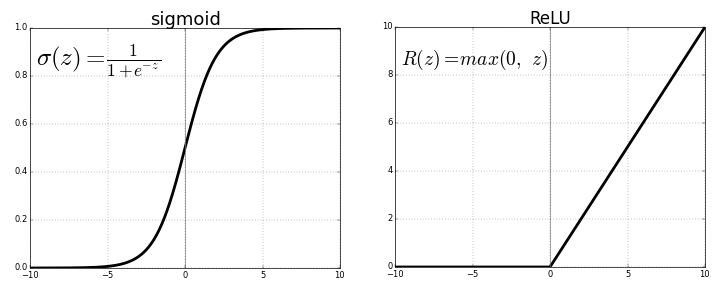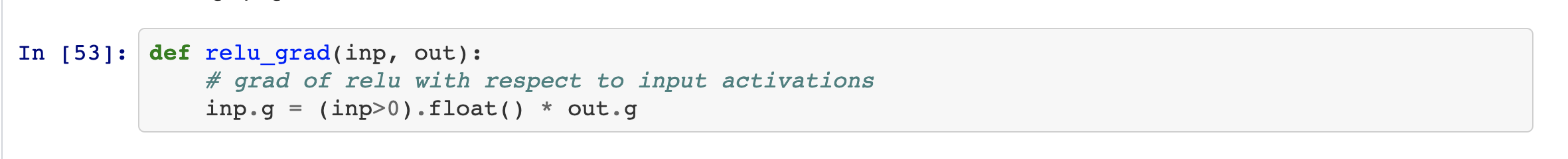$\frac{\partial}{\partial u}MSE(u,y) \times \frac{\partial}{\partial v}l_2(v) \times \color{red}{\frac{\partial}{\partial t}ReLU(t)}\times\frac{\partial}{\partial x}l_1(x)$
def relu_grad(inp, out):
# grad of relu with respect to input activations
inp.g = (inp>0).float() * out.g

• Examplified below
lin1=model_ping('l1') #get value from forward model
lin1.g = (lin1>0).float() * lin2.g;
lin1.g.shape
>>> torch.Size([50000, 50])


4) Derivative of linear1

• Same process with 2) but, this process’s weight has
$\frac{\partial}{\partial u}MSE(u,y) \times \frac{\partial}{\partial v}l_2(v) \times \frac{\partial}{\partial t}ReLU(t)\times\color{red}{\frac{\partial}{\partial x}l_1(x)}$
def lin_grad(inp, out, w, b):
# grad of matmul with respect to input
inp.g = out.g @ w.t()
w.g = (inp.unsqueeze(-1) * out.g.unsqueeze(1)).sum(0)
b.g = out.g.sum(0)

• Examplified below
x_train.g = lin1.g @ w1.t();
w1.g = (x_train.unsqueeze(-1) * lin1.g.unsqueeze(1)).sum(0);
b1.g = lin1.g.sum(0);

x_train.g.shape, w1.g.shape, b1.g.shape
>>> torch.Size([50000, 784])torch.Size([784, 50])torch.Size()


5) Then it goes backward pass

def forward_and_backward(inp, targ):
# forward pass:
l1 = inp @ w1 + b1
l2 = relu(l1)
out = l2 @ w2 + b2
# we don't actually need the loss in backward!
loss = mse(out, targ)

# backward pass:
mse_grad(out, targ)
lin_grad(l2, out, w2, b2)
relu_grad(l1, l2)
lin_grad(inp, l1, w1, b1)


Version 1 (Basic)- Wall time: 1.95 s

Summary

• Notice that output of function at forward pass became input of backward pass
• backpropagation is just the chain rule
• value loss (loss=mse(out,targ)) is not used in gradient calcuation.
• Because, it doesn’t appear with the weight.
• w1g, w2g, b1g, b2g, ig will be used for optimizer
##### check the result using Pytorch autograd
• require_grad_ is the magical function, which can automatic differentiation.2
• This magical auto gradified tensor keep track what happend in forward (taking loss function),
• and do the backward3
• So it saves our time to differentiate ourselves
• Postfix underscore means in pytorch, in-place function, What is in-place function?

⤵️ THis is benchmark…..

Version 2 (torch autograd)- Wall time: 3.81 µs

### Refactor model

• Amazingly, just refactoring our main pieces, it comes down up to Pytorch package.

🌟 Implement yourself, Practice, practice, practice! 🌟

#### Layers as classes

• Relu and Linear are layers in oue neural net. -> make it as classes

• For the forward, using __call__ for the both of forward & backward. Because ‘call’ means we treat this as a function.

class Lin():
def __init__(self, w, b): self.w,self.b = w,b

def __call__(self, inp):
self.inp = inp
self.out = inp@self.w + self.b
return self.out

def backward(self):
self.inp.g = self.out.g @ self.w.t()
# Creating a giant outer product, just to sum it, is inefficient!
self.w.g = (self.inp.unsqueeze(-1) * self.out.g.unsqueeze(1)).sum(0)
self.b.g = self.out.g.sum(0)

• Remember that in lin_grad function, we save bias&weight!!!!!

💬 inp.g : gradient of the output with respect to the input. {: style=”color:grey; font-size: 90%; text-align: center;”}
💬 w.g : gradient of the output with respect to the weight. {: style=”color:grey; font-size: 90%; text-align: center;”}
💬 b.g : gradient of the output with respect to the bias. {: style=”color:grey; font-size: 90%; text-align: center;”}

class Model():
def __init__(self, w1, b1, w2, b2):
self.layers = [Lin(w1,b1), Relu(), Lin(w2,b2)]
self.loss = Mse()

def __call__(self, x, targ):
for l in self.layers: x = l(x)
return self.loss(x, targ)

def backward(self):
self.loss.backward()
for l in reversed(self.layers): l.backward()

• refer to Jeremy’s Model class, he put layers in list

• Dionne’s self-study note: Decomposing Jeremy’s Model class
1. init needs weight, bias but not x data
2. when call that class(a.k.a function) it gave x data and y label!
3. jeremy composited function in layers. x = l(x) so concise…..
4. also utilized that layer list when backward ust reversing it (using python list’s method)
• And he is recursively calling the function on the result of the previous thing. ⬇️
for l in self.layers:
x = l(x)


Q2: Don’t I need to declare magical autograd function, requires_grad_?{: style=”color:red; font-size: 130%; text-align: center;”}

[The questions migrated to this article]

Version 3 (refactoring - layer to class)- Wall time: 5.25 µs

#### Modue.forward()

1. Duplicate code makes execution time slow.
• Role of __call__ changed. No more __call__ for implementing forward pass.
• By initializing the forward with __call__, Module.forward() use overriding to maximize reusability. So any layer inherit Module, can use parent’s function.
2. gradient of the output with respect to the weight
(self.inp.unsqueeze(-1) * self.out.g.unsqueeze(1)).sum(0)


can be reexpressed using einsum,

torch.einsum("bi,bj->ij", inp, out.g)

• Defining forward and Module enables Pytorch to out almost duplicates

Version 4 (Module & einsum)- Wall time: 4.29 µs

Q2: Isn’t there any way to use broadcasting? Why we should use outer product?{: style=”color:red; font-size: 130%; text-align: center;”}

#### Without einsum

Replacing einsum to matrix product is even more faster.

torch.einsum("bi,bj->ij", inp, out.g)


can be reexpressed using matrix product,

inp.t() @ out.g


Version 5 (without einsum)- Wall time: 3.81 µs

#### nn.Linear and nn.Module

Torch’s package nn.Linear and nn.Module

Version 6 (torch package)- Wall time: 5.01 µs

• Final, Using torch.nn.Linear & torch.nn.Module ~~~python

class Model(nn.Module): def init(self, n_in, nh, n_out): super().init() self.layers = [nn.Linear(n_in,nh), nn.ReLU(), nn.Linear(nh,n_out)] self.loss = mse

def __call__(self, x, targ):
for l in self.layers: x = l(x)
return self.loss(x.squeeze(), targ)


class Model(): def init(self): self.layers = [Lin(w1,b1), Relu(), Lin(w2,b2)] self.loss = Mse()

def __call__(self, x, targ):
for l in self.layers: x = l(x)
return self.loss(x, targ)

def backward(self):
self.loss.backward()
for l in reversed(self.layers): l.backward()


~~~